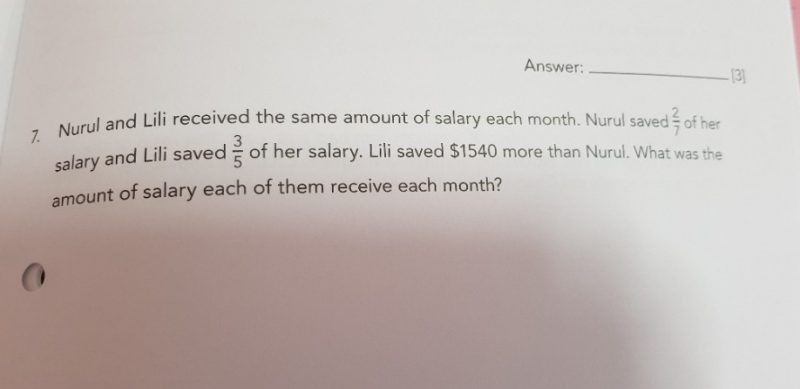QuestionP5 section c paper 2. Please help. Thank you so much😊

Both earned the same salary, meaning the bars in the model are the same length. Find the LCM for the denominators in order to compare the difference in value of the savings with the units.

LCM for 7 and 5 is 35.

2X 5=10 (Nurul saves 10 units)

3×7= 21 (Lili saves 21 units)

21 – 10 = 11 (difference in units)

1540÷11=140 (value of 1 unit)

140×35 =4900 (Value of 35 units)

And is \$4900

0 Replies 0 Likes

Thank you for explaining on sunday😉

0 Replies 0 Likes